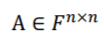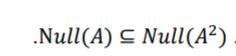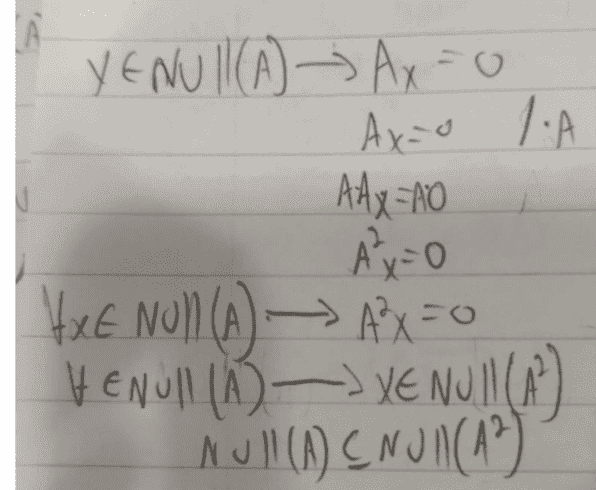Null Space of a matrix

Homework Statement

givenI am required to proove or disprove:[/B]rank
dim
null space

The Attempt at a Solution

I tried to base my answer based on the fact that null A and null A^2 is Contained in F (n)
and
dim N(A)+rank(A)=N
same goes for A^2.

Attachments

member 587159
Why don't you just use the definition?

##x \in Null(A) \implies Ax = 0 ##

If the statement is true, you have to prove that ##A^2 x = 0##. Can you show that?

•sarumman
Why don't you just use the definition?

##x \in Null(A) \implies Ax = 0 ##

If the statement is true, you have to prove that ##A^2 x = 0##. Can you show that?
thank you! you mean like so:Attachments

•WWGD
member 587159
Yes, the idea is certainly correct. The proof exposition can be better though. Here is how I would write it:

We want to prove that ##Null(A) \subseteq Null(A^2)##, so let's take an arbitrary element ##x \in Null(A)##. By definition, this means that ##Ax = 0##. Since ##A^2x = (AA)x = A(Ax) = A0 = 0## (here we used associativity of matrix multiplication/function composition), it follows that ##x \in Null(A^2)##, and we are done.

•FactChecker and WWGD
PeterDonis
Mentor